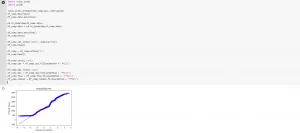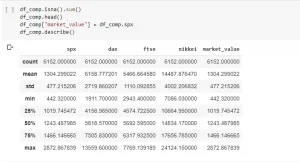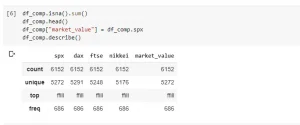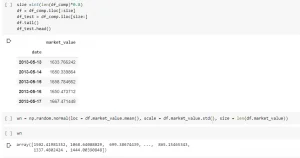09 Apr 2020

Posted on:

06 Apr 2020

0

# white noise

wn = np.random.normal(loc = df.market_value.mean(), scale = df.market_value.std(), size = len(df))
```while Running this code i was getting this errorNote: My code editor is Google colab---------------------------------------------------------------------------
```
```TypeError                                 Traceback (most recent call last)
```
```<ipython-input-70-e07c336ccb3d> in <module>()
----> 1 wn = np.random.normal(loc = df.market_value.mean(), scale = df.market_value.std(), size = len(df))

```
5 frames
```/usr/local/lib/python3.6/dist-packages/numpy/core/_methods.py in _sum(a, axis, dtype, out, keepdims, initial, where)
36 def _sum(a, axis=None, dtype=None, out=None, keepdims=False,
37          initial=_NoValue, where=True):
---> 38     return umr_sum(a, axis, dtype, out, keepdims, initial, where)
39
40 def _prod(a, axis=None, dtype=None, out=None, keepdims=False,

```
`TypeError: unsupported operand type(s) for +: 'float' and 'str'`
Instructor
Posted on:

07 Apr 2020

0

Hey Ramanjaneyulu,

Thanks for reaching out!

I've never had this specific error happen to me before. Would you mind letting me know what versions of pandas and NumPy you're using here? Without that, I can give only 1 suggestion - try putting all the values apart from size into 'float()', like so:

wn = np.random.normal(loc = float(df.market_value.mean()), scale = float(df.market_value.std()), size = len(df))

Best
365 Vik

Instructor
Posted on:

09 Apr 2020

0

Hello again, Ram!

1.) It is crucial that you reload the data. If you've already filled it with text, you won't find any new missing elements to fill properly. (Easiest thing to do is just rerun the entire code - top to bottom.)

2.) You need to fill the missing values in SPX before assigning its values to market_value. Otherwise, you're not changing the variable you're using to create the random walk.

3.) You need to change method = "ffill" in 4 separate places.4.) If you've done this correctly, when you describe the dataframe your table should look like so:If you've done it the way you did earlier, you're going to have "ffill" as the top value.5.) If you've made the changes appropriately, the code will run smoothly and you'll get an output for the white noise variable.For good measure, here is a link to my Google Colabs file, so you can cross-reference your code (I mostly just copied the code you sent me earlier).

Best,
365 Vik